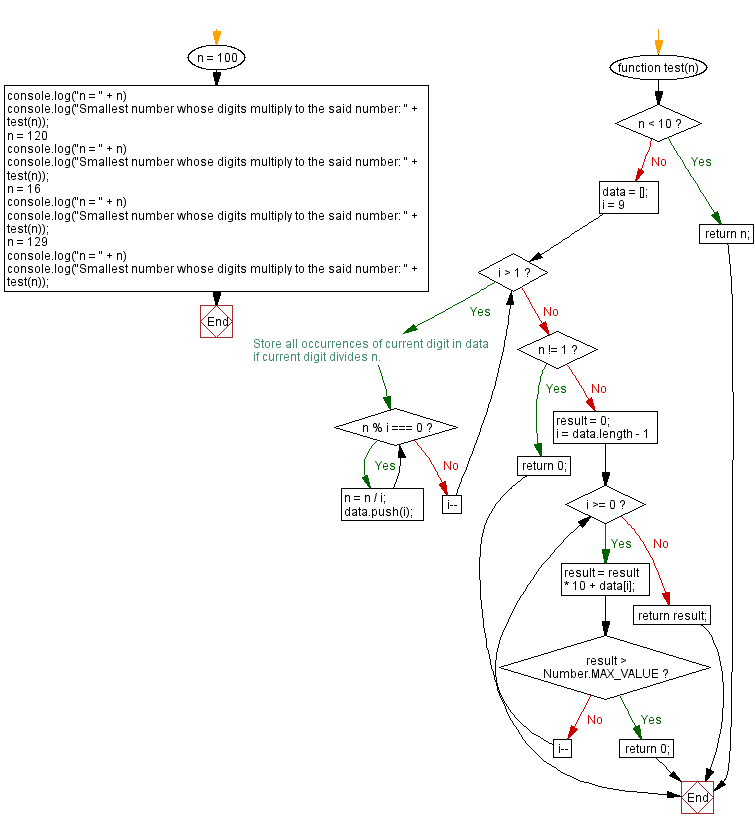# JavaScript: Smallest number whose digits multiply to a number

## JavaScript Math: Exercise-113 with Solution

Write a JavaScript program to calculate the smallest number whose digits multiply into a given number.

Example:
let n =100

Test Data:
(100) -> 455
(120) -> 358
(16) -> 28
(129) -> 0

Sample Solution:

HTML Code:

``````<!DOCTYPE html>
<html>
<meta charset="utf-8">
<title>JavaScript program to Smallest number whose digits multiply to a number</title>
<body>

</body>
</html>
```
```

JavaScript Code:

``````function test(n) {
if (n < 10) return n;
data = [];
for (i = 9; i > 1; i--) {
// Store all occurrences of current digit in data
// if current digit divides n.
while (n % i === 0) {
n = n / i;
data.push(i);
}
}

if (n != 1) return 0;
result = 0;
for (i = data.length - 1; i >= 0; i--) {
result = result * 10 + data[i];
if (result > Number.MAX_VALUE) return 0;
}
return result;
}
n = 100
console.log("n = " + n)
console.log("Smallest number whose digits multiply to the said number: " + test(n));
n = 120
console.log("n = " + n)
console.log("Smallest number whose digits multiply to the said number: " + test(n));
n = 16
console.log("n = " + n)
console.log("Smallest number whose digits multiply to the said number: " + test(n));
n = 129
console.log("n = " + n)
console.log("Smallest number whose digits multiply to the said number: " + test(n));
```
```

Sample Output:

```n = 100
Smallest number whose digits multiply to the said number: 455
n = 120
Smallest number whose digits multiply to the said number: 358
n = 16
Smallest number whose digits multiply to the said number: 28
n = 129
Smallest number whose digits multiply to the said number: 0
```

Flowchart:Live Demo:

See the Pen javascript-math-exercise-113 by w3resource (@w3resource) on CodePen.

Improve this sample solution and post your code through Disqus

What is the difficulty level of this exercise?

Test your Programming skills with w3resource's quiz.

﻿